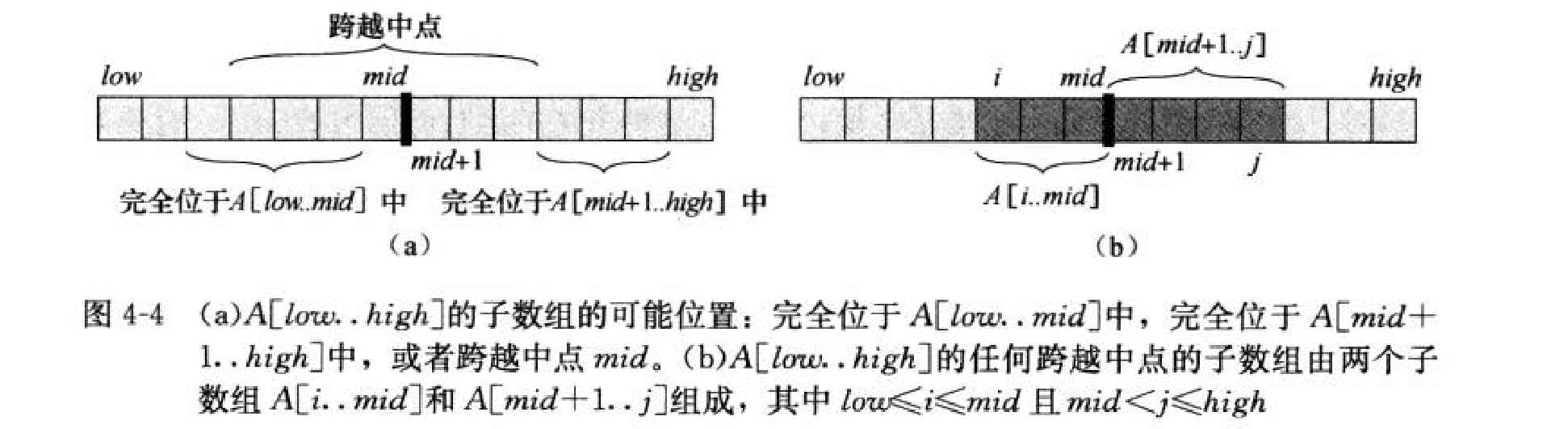# 最大连续子数组--分治法

分治解法：

对于一个数组 A [ low..high ] , 我们可以将其划分为两个规模相等的子数组A [ low..mid ] 和 A [ mid+1..high ]，其中 mid=(low+high)/2。那么，所有的连续子数组必定位于以下三种位置之一：

（1）完全位于左子数组中：

（2）完全位于右子数组中：

（3）跨越中点：因此，我们可以设计一个分治算法来求解这一问题：

（1）分解

如上所述。

（2）求解

当 数组分解到只有一个元素时，那么此时这个必然是最大的连续数组，直接返回这个数组。判断条件便为 low == high。

（3）合并

取 左子数组的最大值、右子数组的最大值、跨越中点的数组的最大值  三者的最大值。

其中，跨越中点的数组的最大值，需要 O(n) 扫一遍数组。即对每一个递归求解的数组， 先扫一遍中点左边，求出以mid为后缀的 left-max ； 然后扫一遍中点右边，求出以 mid+1 的点为前缀的 right-max，最后返回 （left-max）+（right-max），两者之和即为跨越中点的最大值。

class Solution {
public:
//分治法
//合并的部分，注意返回的值是左边加右边的和
int max_cross_subArray(vector<int> &nums,int low,int mid,int high)
{
int left_sum=INT_MIN,right_sum=INT_MIN;
int sum=0;
for(int i=mid;i>=low;i--){
sum+=nums[i];
left_sum=max(left_sum,sum);
}
sum=0;
for(int i=mid+1;i<=high;i++){
sum+=nums[i];
right_sum=max(right_sum,sum);
}
return left_sum+right_sum;
}
//分治
int max_subArray(vector<int>&nums,int low,int high)
{
if(low==high){
return nums[low];
}

int mid=(low+high)/2;

int left_sum=max_subArray(nums,low,mid);
int right_sum=max_subArray(nums,mid+1,high);

int cross_sum=max_cross_subArray(nums,low,mid,high);
//max
return max(max(left_sum,right_sum),cross_sum);
}

int maxSubArray(vector<int>& nums) {
int ans=nums;
int left_pos=0,right_pos=0;
int pre_sum=ans,pre_left=0;

//分治法
ans=max_subArray(nums,0,nums.size()-1);
return ans;

}
};
posted @ 2020-01-13 14:57  Litn  阅读(262)  评论(0编辑  收藏